# Numerical Integration### Analytical Vs. Numerical

A differential equation that models the behavior of a neuron was developed earlier. It is a powerful system that allows for a good deal of dynamic behavior that emulates the actions of real neurons quite nicely. However, that leaves a rather large problem of how do you actually use that equation. It is reasonably complex and dependant on some non-linear inputs. Finding an analytical solution to this equation would not be a simple thing. In other words, it would be very difficult to crack open your college calculus book and try and derive a complete differential equation and solve it. The reason for this is that in order to write the equation, the equations for all input and output systems must also be known. And those input and output systems can be extremely complex, using random variables and discontinuous and nonlinear functions. Luckily, numerical methods exists that allow the differential equation to be solved without having to actually solve an equation analytically or worry about all of those complex systems. The numerical method used here is the Euler method. In order to understand the Euler method for solving differential equations it is necessary to understand some basics about Taylor series.

### Taylor Series

If a function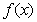has continuous derivatives up to (n+1)th order, then this function can be expanded in the following fashion: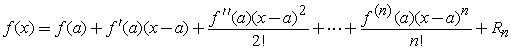(1)

where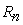, called the remainder after n+1 terms, is given by: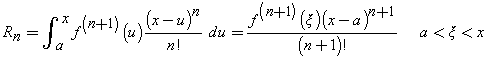(2)

When this expansion converges over a certain range of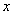, that is,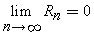, then the expansion is called the Taylor Series ofexpanded about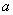. If the value of (x-a) is then replaced with (x-a) = h in equation 1 then we end up with equation 3.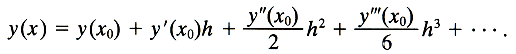(3)

The important thing to remember about the Taylor series is that it is a way to find the value of a function at a specific value of x when you do not actually have a formal equation defining the original function y(x). Instead, all you need is the value of the function at some starting point like time zero, and you need the first derivative and second derivative and so on. This simplifies the problem greatly because it is often much easier to take derivatives of a function than it is to find the integral of that function. And in this case the equation that we currently have is the one for the first derivative.

### 3. Euler Method

The Euler method simplifies the Taylor equations even farther. It eliminates the need to find all of those other derivatives. It is known that the error in the Taylor series will be small if the step size h is small. If the step size is made small enough then only a few terms are needed in order to solve the differential equation with good accuracy. The Euler method follows this idea to the extreme for first order differential equations. It uses only the first two terms of the Taylor series! This then gives the equation in 4 for the Euler method where the O(h2) term represents the error that occurs because of this simplifying assumption.(4)

An example is probably in order here to help explain exactly what is going on in more detail. Equation 5 gives a sample first order differential equation and lists the starting value of y(0), and h = 0.1. With this information it is possible to generate a table that lists the values of the important variables for the first five iterations of x. These values are listed in table 1. The analytical solution to equation 5 is given in equation 6. A few moments spent playing around with a calculator will show that the Euler method is giving values reasonably close to those from the analytical solution. And to get values that are even closer, then all that needs to be done is to use a smaller value of h.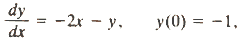(5)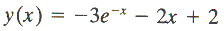(6)

 Table 1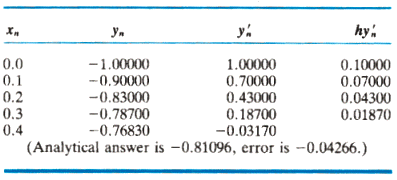So the really important thing that should be taken from all of this is that by using Euler's method for solving first order differential equations it is possible to make a simple algorithm that takes the current value for VN, adds up all of the all of the values in the model equation to get a value for V'N, and then uses equation 4 to get a new value for the membrane potential. This is the basics of how the neural models in this plug-in do their calculations.